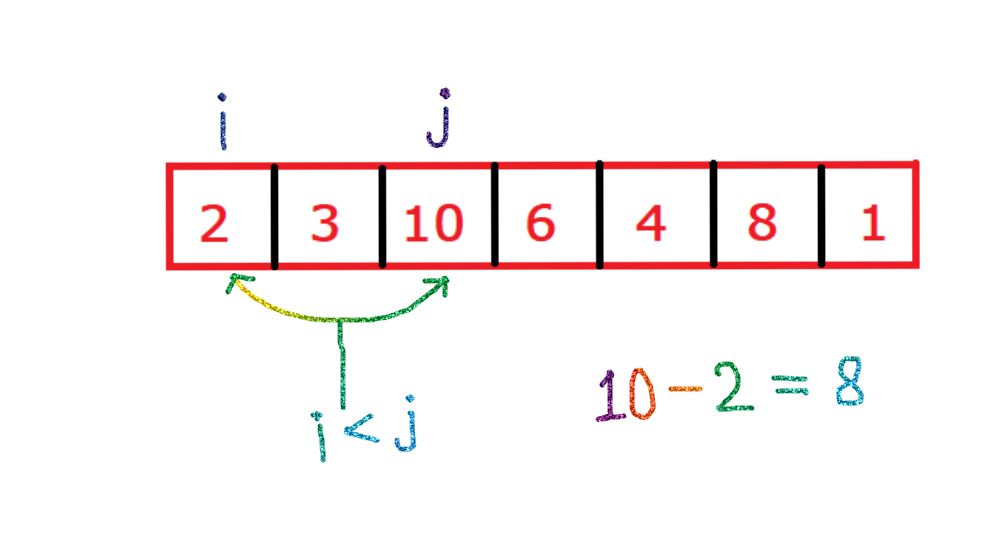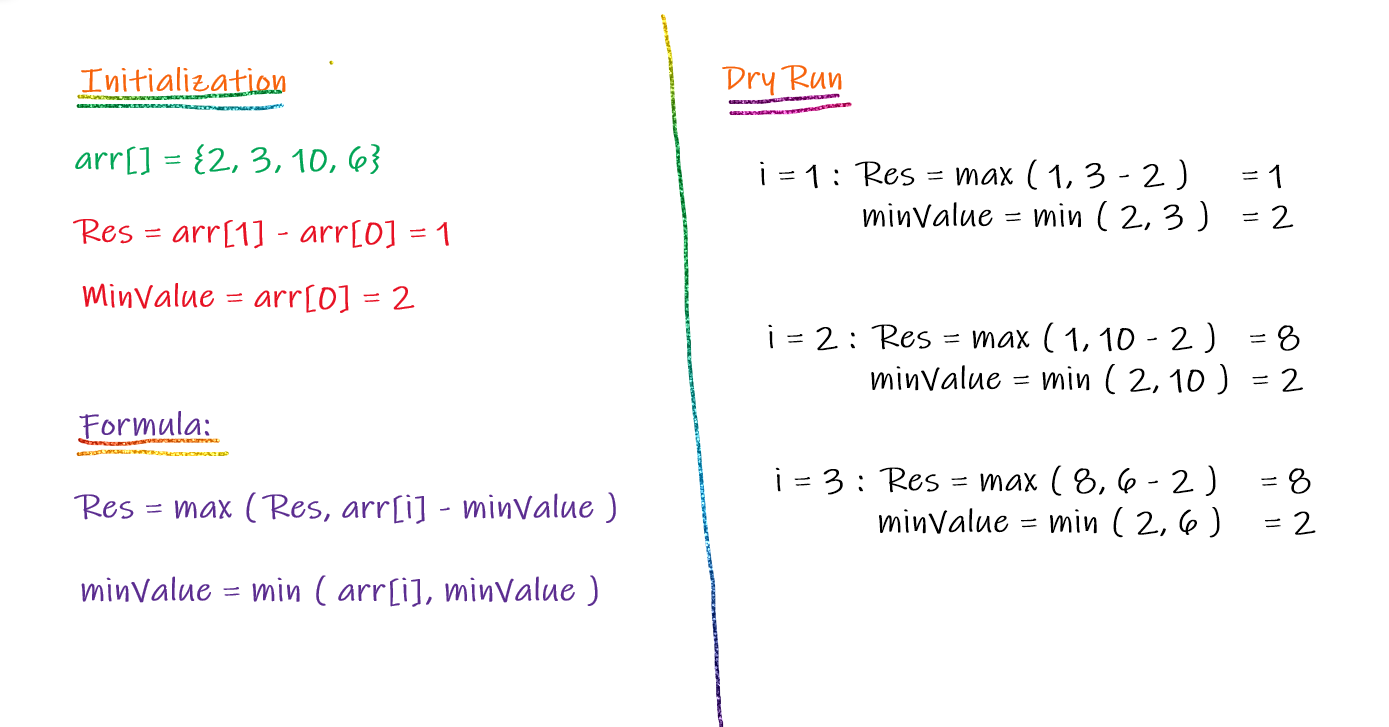# Maximum Difference Problem with Order

Problem Statement: Given an array of integers of size N, Our task is to find the maximum difference between two numbers such that (i < j) i.e Order should be maintained.

Examples:

```Example 1:
Input: N = 7, array[] = {2, 3, 10, 6, 4, 8, 1}
Output: 8
Explanation: Here the maximum element is 10 and the
minimum element is 2, Both elements satisfy the
condition (i < j), So the difference between maximum
and minimum is always maximum so the answer
is (10 - 2) = 8.Example 2:
Input: N = 5, array[] = {100, 1, 2, 5, 100}
Output: 99
Explanation: Here the maximum element is 100 and
the minimum element is 1, Both elements satisfy
the condition (i < j), So the difference between
maximum and minimum is always maximum so the
answer is (100 - 1) = 8.```

Disclaimer: Don’t jump directly to the solution, try it out yourself first.

### Solution 1: Naive Solution

• We will use two nested loops.
• The outer loop will help to pick up elements one by one.
• The inner loop starts from the next index of the picked element. Calculate the difference of the picked element with every other element in the array.
• Compare the difference with the maximum difference current maximum difference calculated so far.
• At the end return, the maximum difference is calculated.

Code:

## C++ Code

``````#include<bits/stdc++.h>

using namespace std;

int maxDiff(int arr[], int n) {
int res = arr - arr;

for (int i = 0; i < n - 1; i++) {
for (int j = i + 1; j < n; j++) {
res = max(res, arr[j] - arr[i]);
}
}

return res;
}

int main() {

int arr[] = {2, 3, 10, 6, 4, 8, 1}, n = 7;

cout << "The maximum difference with order is: " << maxDiff(arr, n);
}``````

Output: The maximum difference with order is: 8

Time Complexity: O(N^2)

Space Complexity: O(1)

## Java Code

``````class TUF {

static int maxDiff(int arr[], int n) {
int res = arr - arr;

for (int i = 0; i < n - 1; i++) {
for (int j = i + 1; j < n; j++) {
res = Math.max(res, arr[j] - arr[i]);
}
}

return res;
}

public static void main(String args[]) {
int arr[] = {2,3,10,6,4,8,1}, n = 7;

System.out.println("The maximum difference with order is: " + maxDiff(arr, n));

}

}``````

Output: The maximum difference with order is: 8

Time Complexity: O(N^2)

Space Complexity: O(1)

### Solution 2: Efficient Solution (Single traversal)

• In this approach, we will keep track of two things.
• First, We will keep track of the Maximum difference so far.
• Second, We will keep track of the minimum element we have found so far.
• So, we will initialize the maximum difference as arr[start+1] – arr[start].
• And for the minimum value that will be initialized as arr[start].
• If arr[i] – minimum value is greater than the maximum difference so far, then we will update the maximum difference with this new value.
• If arr[i] is less than the minimum element we found so far then we will update the minimum value with arr[i].
• After traversing the array in the above manner at last we will be left with the maximum difference with the order.
• Print / Return it.

### Dry Run Implementation:Code:

## C++ Code

``````#include <bits/stdc++.h>

using namespace std;

int maxDiff(int arr[], int n) {
int res = arr - arr, minVal = arr;

for (int i = 1; i < n; i++) {

res = max(res, arr[i] - minVal);

minVal = min(minVal,arr[i]);
}

return res;
}

int main() {

int arr[] = {2, 3, 10, 6, 4, 8, 1}, n = 7;

cout << "The maximum difference with order is: " << maxDiff(arr, n);

}``````

Output:

Output: The maximum difference with order is: 8

Time Complexity: O(N)

Space Complexity: O(1)

## Java Code

``````class TUF {

static int maxDiff(int arr[], int n) {
int res = arr - arr, minVal = arr;

for (int i = 1; i < n; i++) {

res = Math.max(res, arr[i] - minVal);

minVal = Math.min(minVal, arr[i]);
}

return res;
}

public static void main(String args[]) {
int arr[] = {2, 3, 10, 6, 4, 8, 1}, n = 7;

System.out.println("The maximum difference with order is : " + maxDiff(arr, n));

}

}``````

Output:

Output: The maximum difference with order is: 8

Time Complexity: O(N)

Space Complexity: O(1)

Special thanks to Abhishek Yadav for contributing to this article on takeUforward. If you also wish to share your knowledge with the takeUforward fam, please check out this article